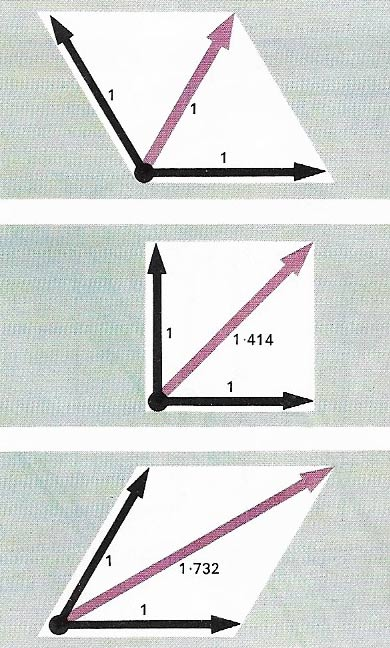A# parallelogramParallelogram of forces. Two forces act simultaneously on an object in different directions. The resultant force, and the direction of any subsequent movement, is defined by the diagonal of a parallelogram whose sides are drawn parallel to the applied forces with lengths proportional to their magnitudes. This is an application of vector diagrams.

A parallelogram is a quadrilateral (four-sided figure) whose opposite sides are parallel, and whose opposite angles, therefore, are equal. The diagonals of a parallelogram bisect each other. A parallelogram of base b and height h has an area:

A = bh = ab sinA = ab sinB.

The height of a parallelogram is h = a sinA = a sinB. The sides a, b, c, and d and diagonals p and q of a parallelogram satisfy the equality:

p 2 + q 2 = a 2 + b 2 + c 2 + d 2

Special cases of a parallelogram are a rhombus, which has sides of equal length, a rectangle, which has two sets of parallel sides that are perpendicular to each other, and a square, which meets the conditions of both a rectangle and a rhombus.

## Parallelogram of forces

If a particle is under the action of two forces, which are represented in magnitude and direction by the two sides of a parallelogram drawn from a point, the resultant of the two forces is represented by the diagonal of the parallelogram drawn from that point.

## Parallelogram of velocities

If a body has two component velocities, represented in magnitude and direction by two adjacent sides of a parallelogram drawn from a point, the resultant velocity of the body is represented by the diagonal of the parallelogram drawn from that point. The parallelogram of velocities, like the parallelogram of forces, is a particular case of the parallelogram of vectors.

A familiar application of it is to find the resultant velocity of an object, such as a boat, that is subject to a current acting in a different direction in the one in which it is trying to move.

## Zonohedron

A zonohedron is a polyhedron in which the faces are all parallelograms or parallel-sided. The faces of a zonohedron can be grouped into zones – encircling bands of faces which share a common edge direction (and length).